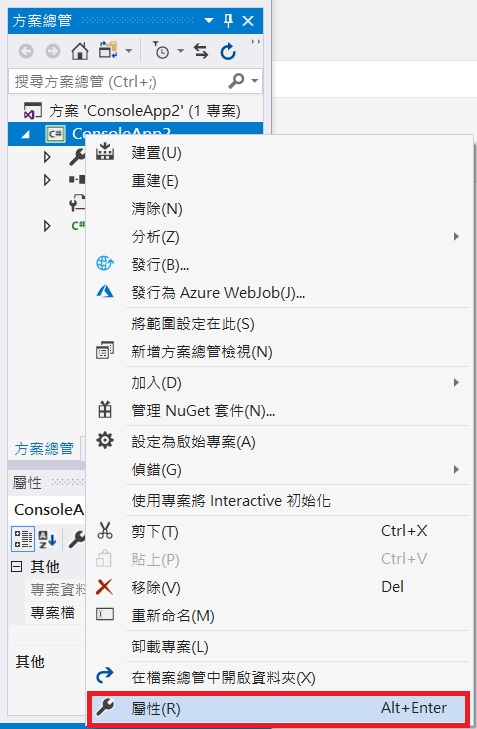# 函數(function)

class Program
{
static void Main(string[] args)
{
int[] r = { 10, 15, 20, 30 };
for (int i = 0; i < r.Length; i++)
{
Console.WriteLine("半徑:{0}, 圓面積 : {1}", r[i], CircleArea(r[i]));
}
}
static double CircleArea(int r)
{
return Math.PI * r * r;
}
}


# 語法

1. 上面少了static這個字, 因這個觀念比較困難, 留待物件導向時再說明, 現在只需記得在程式中一定要加static
2. 每個函數最多只能有一個返回值, 也可以沒有返回值. 當沒有返回值時, 需填入 void
3. 函數名稱需作整体的規畫, 方便日後的維護管理
4. 參數可以有多個, 也可以沒有參數

# 函數原型宣告

#include <iostream>
using namespace std;
double circle_area(int); //函數原型宣告
int main(){
printf("半徑 : %d, 圓面積 : %f", 10, circle_area(10));
}

//底下是函數主体區
double circle_area(int r){
return 3.14159*r*r;
}

Main中的 x:5, y:5



# Call by value(傳值呼叫)

 class Program
{
static void Main(string[] args)
{
int x = 5, y = 10;
Console.WriteLine("Main中的 x:{0}, y:{0}", x, y);
}
static int Add(int x, int y)
{
x = x * 10;
y = y * 10;
return x + y;
}
}

Main中的 x:5, y:5

# Call by reference(傳參考呼叫)

namespace ConsoleApp2
{
class Program
{
static void Main(string[] args)
{
Pikachu p = new Pikachu();
p.level = 10;
Console.WriteLine("Main中的 p.level : {0}", p.level);
}
{
x.level = 100;
}
}
class Pikachu
{
public int level;
}
}

Main中的 p.level : 100



    class Program
{
static void Main(string[] args)
{
int i=10;
Console.WriteLine("Main中的 i: {0}", i);
}
{
i = 100;
}
}


class Program
{
static void Main(string[] args)
{
int []array= {1,2,3,4,5 };
for (int i = 0; i < array.Length; i++)
{
Console.WriteLine("Main中的 array[{0}]:{1}", i, array[i]);
}

}
{
for (int i = 0; i < array.Length; i++)
{
array[i] = array[i] * 10;
}

}
}

Main中的 array:10
Main中的 array:20
Main中的 array:30
Main中的 array:40
Main中的 array:50class Program
{
static unsafe void Main(string[] args)
{
int i=10;
Console.WriteLine("Main中的 i: {0}", i);
}
{
*i = 100;
}
}


C#不建議使用指標, 所以稱為不安全的程式碼. 所以盡量少用. 可以使用委派(delegate)取代指標

# 傳出參數

class Program
{
static void Main(string[] args)
{
int i;
Console.WriteLine("Main中的 i: {0}", i);
}
{
i = 10;
}
}

Main中的 i: 10

class Program
{
static void Main(string[] args)
{
int x = 10;
int y = 20;
float z = 20;
int m=Sum(x, y);
float n = Sum(x, z);
Console.WriteLine("m={0}", m);
Console.WriteLine("n={0}", n);
}
static int Sum(int x, int y)
{
Console.WriteLine("現在跑第一個 Sum");
return x + y;
}
static float Sum(int x, float y)
{
Console.WriteLine("現在跑第二個 Sum");
return x + y;
}
}


static void Sum(int x, int y), 下面幾種寫法請仔細參照一下

static void Sum(int a, int b) : 簽名相同, 不能重載
static void Sum(int x, int y, int z) : 數量不同, 所以簽名不同, 可以重載
static void Sum(int x, float y) : 參數型態不同, 所以簽名不同, 可以重載
static int Sum(int a, int b) : 簽名不包含傳回值, 所以不可重載

# 變數總類

class Program
{
static int a = 10;//類別變數
static void Main(string[] args)
{
int b = 20;//Main的區域變數
{
int c = 30; //區塊變數
}
Console.WriteLine("a={0}", a);
//Console.WriteLine("c={0}", c); c 離開區塊就死亡, 所以不能在此使用
//Console.WriteLine("d={0}", d); d 是別人家的孩子, 不可以随便亂打

}
static int Sum(int x, int y)
{
Console.WriteLine("a={0}", a);
int d = 40;
return x + y;
}
}


a : 前面加上static, 稱類別變數, 其效力可達Main, Add, 也就是凡是在Class內, 都可以使用
b : Main的區域變數, 只能在Main中使用
c : Main的區塊變數, 一離開區塊就立即死亡
1.Jolyn Orsburn